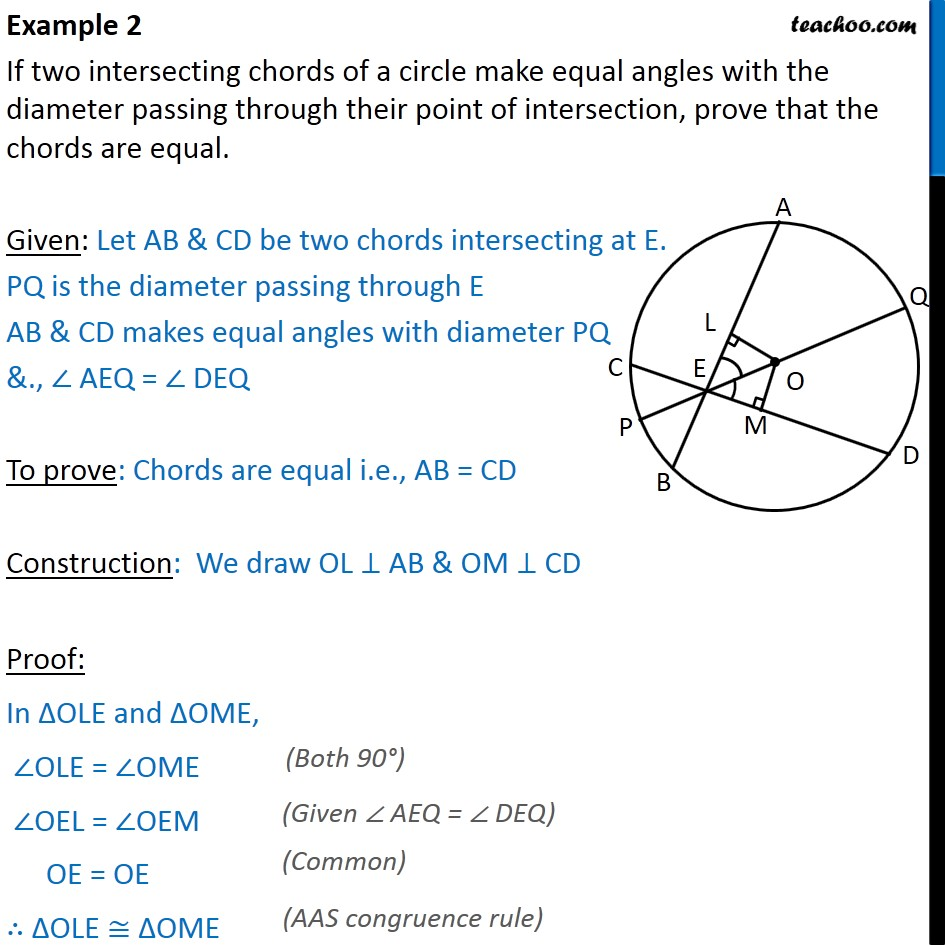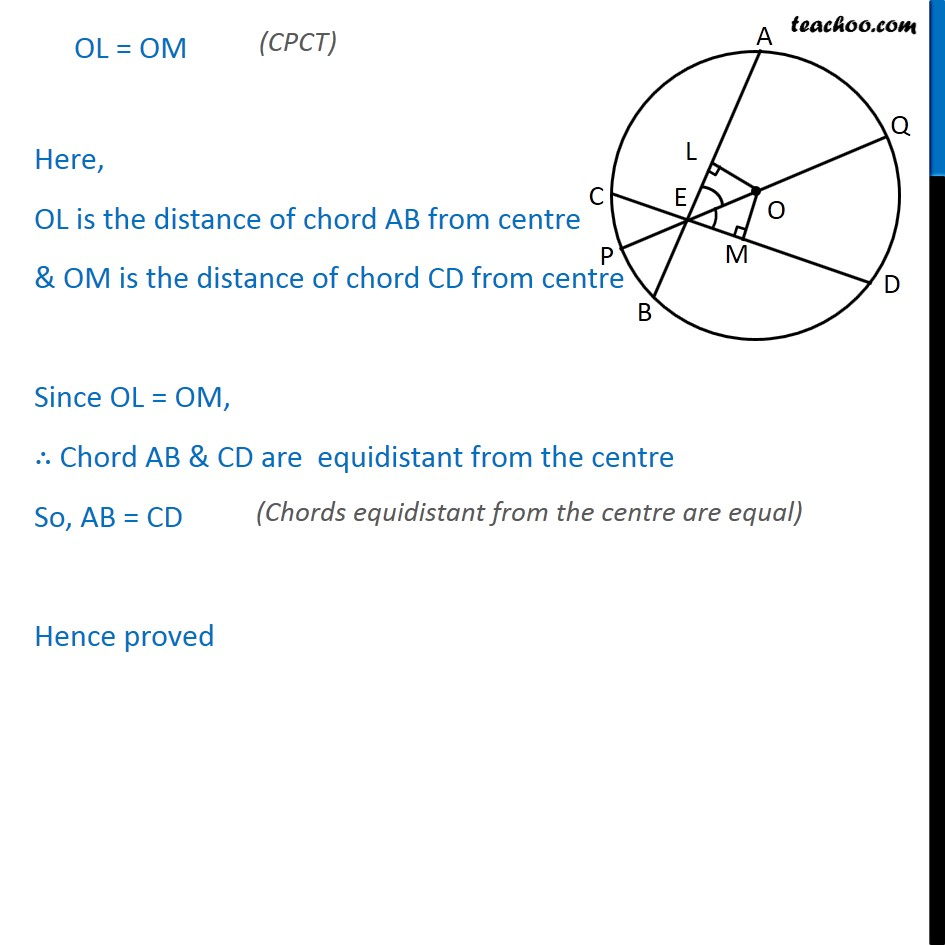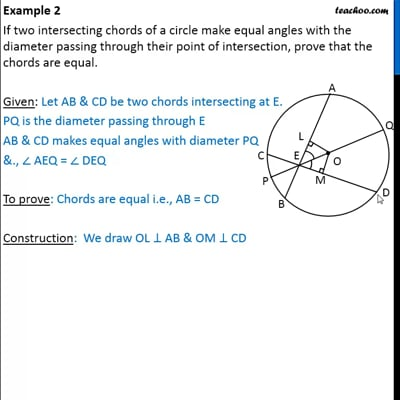Examples

Chapter 10 Class 9 Circles
Serial order wiseThis video is only available for Teachoo black users

Maths Crash Course - Live lectures + all videos + Real time Doubt solving!

### Transcript

Example 2 If two intersecting chords of a circle make equal angles with the diameter passing through their point of intersection, prove that the chords are equal. Given: Let AB & CD be two chords intersecting at E. PQ is the diameter passing through E AB & CD makes equal angles with diameter PQ &., AEQ = DEQ To prove: Chords are equal i.e., AB = CD Construction: We draw OL AB & OM CD Proof: In OLE and OME, OLE = OME OEL = OEM OE = OE OLE OME OL = OM Here, OL is the distance of chord AB from centre & OM is the distance of chord CD from centre Since OL = OM, Chord AB & CD are equidistant from the centre So, AB = CD Hence proved